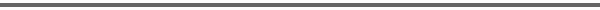Journal Home Page Complete Contentsof this Volume Previous Article Next Article Journal of Lie Theory 14 (2004), No. 2, 509--522 Copyright Heldermann Verlag 2004Injectivity of the Double Fibration Transform for Cycle Spaces of Flag Domains Alan T. Huckleberry Fakultät für Mathematik, Ruhr-Universität, 44780 Bochum, Germany, ahuck@cplx.ruhr-uni-bochum.de Joseph A. Wolf Dept. of Mathematics, University of California, Berkeley CA 94720-3840, U.S.A., jawolf@math.berkeley.edu[Abstract-pdf] The basic setup consists of a complex flag manifold $Z=G/Q$ where $G$ is a complex semisimple Lie group and $Q$ is a parabolic subgroup, an open orbit $D = G_0(z) \subset Z$ where $G_0$ is a real form of $G$, and a $G_0$--homogeneous holomorphic vector bundle $\mathbb E \to D$. The topic here is the double fibration transform ${\cal P}: H^q(D; {\cal O}(\mathbb E)) \to H^0({\cal M}_D;{\cal O}(\mathbb E'))$ where $q$ is given by the geometry of $D$, ${\cal M}_D$ is the cycle space of $D$, and $\mathbb E' \to {\cal M}_D$ is a certain naturally derived holomorphic vector bundle. Schubert intersection theory is used to show that ${\cal P}$ is injective whenever $\mathbb E$ is sufficiently negative. [FullText-pdf (238 KB)] for subscribers only.